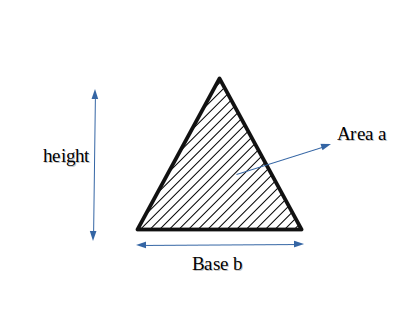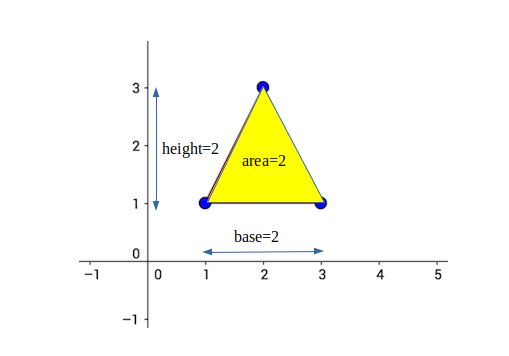# Minimum height of a triangle with given base and area

Given two integers a and b, find the smallest possible height such that a triangle of atleast area “a” and base “b” can be formed.Examples :

```Input : a = 2, b = 2
Output : Minimum height of triangle is 2

Explanation:Input : a = 8, b = 4
Output : Minimum height of triangle is 4
```

## Recommended: Please try your approach on {IDE} first, before moving on to the solution.

Minimum height of Triangle with base “b” and area “a” can be evaluated by having the knowledge of the relationship between the three.

The relation between area, base and
height is:
area = (1/2) * base * height

So height can be calculated as :
height = (2 * area)/ base

Minimum height is the ceil of the
height obtained using above formula.

## C++

 `#include ` `using` `namespace` `std; ` ` `  `// function to calculate minimum height of  ` `// triangle ` `int` `minHeight(``int` `base, ``int` `area){ ` `    ``return` `ceil``((``float``)(2*area)/base); ` `} ` ` `  `int` `main() { ` `    ``int` `base = 4, area = 8; ` `    ``cout << ``"Minimum height is "`  `         ``<< minHeight(base, area) << endl; ` `    ``return` `0; ` `} `

## Java

 `// Java code Minimum height of a  ` `// triangle with given base and area ` ` `  `class` `GFG { ` `     `  `    ``// function to calculate minimum height of  ` `    ``// triangle ` `    ``static` `double` `minHeight(``double` `base, ``double` `area) ` `    ``{ ` `        ``double` `d = (``2` `* area) / base; ` `        ``return` `Math.ceil(d); ` `    ``} ` `     `  `    ``// Driver code ` `    ``public` `static` `void` `main (String[] args)  ` `    ``{ ` `        ``double` `base = ``4``, area = ``8``; ` `        ``System.out.println(``"Minimum height is "``+ ` `                            ``minHeight(base, area)); ` `    ``} ` `} ` `// This code is contributed by Anant Agarwal. `

## Python

 `# Python Program to find minimum height of triangle ` `import` `math ` ` `  `def` `minHeight(area,base): ` `        ``return` `math.ceil((``2``*``area)``/``base) ` ` `  `# Driver code ` `area ``=` `8` `base ``=` `4` `height ``=` `minHeight(area, base) ` `print``(``"Minimum height is %d"` `%` `(height)) `

## C#

 `// C# program to find minimum height of ` `// a triangle with given base and area ` `using` `System; ` ` `  `public` `class` `GFG { ` ` `  `    ``// function to calculate minimum ` `    ``// height of triangle ` `    ``static` `int` `minHeight(``int` `b_ase, ``int` `area) ` `    ``{ ` `        ``return` `(``int``)Math.Round((``float``)(2 * area) / b_ase); ` `    ``} ` `     `  `    ``// Driver function ` `    ``static` `public` `void` `Main() ` `    ``{ ` `        ``int` `b_ase = 4, area = 8; ` `        ``Console.WriteLine(``"Minimum height is "` `                        ``+ minHeight(b_ase, area)); ` `    ``} ` `} ` ` `  `// This code is contributed by vt_m. `

## PHP

 ` `

Output :

```Minimum height is 4
```

Attention reader! Don’t stop learning now. Get hold of all the important DSA concepts with the DSA Self Paced Course at a student-friendly price and become industry ready.

My Personal Notes arrow_drop_upCheck out this Author's contributed articles.

If you like GeeksforGeeks and would like to contribute, you can also write an article using contribute.geeksforgeeks.org or mail your article to contribute@geeksforgeeks.org. See your article appearing on the GeeksforGeeks main page and help other Geeks.

Please Improve this article if you find anything incorrect by clicking on the "Improve Article" button below.

Improved By : vt_m

Article Tags :
Practice Tags :

Be the First to upvote.

Please write to us at contribute@geeksforgeeks.org to report any issue with the above content.